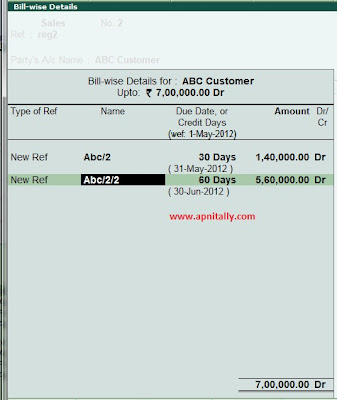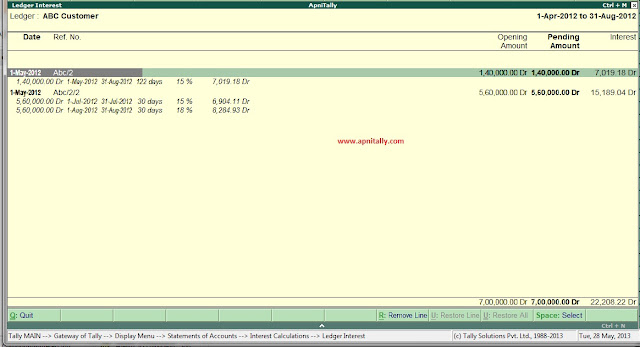# Interest Calculation : How to do it in Tally.ERP 9

In our practical situations in business some times need arises that to curtail the balances due or to keep them to minimum levels we need to charge interest. Some times this also happens that we are charged interests on late payment. In both the situation we need to calculate the interest  accurately.  Interest calculation is a feature in Tally.ERP 9 with many options and choices.This feature is so flexible that you can fit all your requirements related to interest calculation in it.Interest Calculation
With Tally interest calculation feature you can :-
1. Calculate interest on transactions with selective parties.
2. You can calculate interest on desired rate, with desired period, on desired method.
3. You can even calculate interest on single transaction with multiple rates.
4. You can calculate simple interest as well as compound interest.

Activate Interest calculation :
Before starting we have to activate interest calculation in Tally. So to do so we need to go to F11 and then in F1 Accounting features and set Activate interest calculation to Yes. Also set use advance parameters to Yes. Now interest calculation in this company is activated. See the photo for guidelines.Activating Interest calculation
If you have planning to charge interest on each transactions then make sure to change Maintain Bill By Bill Details to Yes also.

Now you have to activate parties on which you want to charge interest. Go to Account Info==> Ledgers==> Alter==> and put Activate Interest Calculation to Yes. Now define your interest parameters here. See photographs to understand each option.Activate Interest calculation on Party.Interest calculation parameters
Here in interest calculation parameters, we have decided to charge interest @ 15% yearly on transaction by transaction on debit balances only, which is applicable past due date would be calculated from due date of invoice/Ref.
Here you can change the rate of interest, You can change rate per month, per 365 days, per calendar year and calendar month.
You can change the applicability based on effective due date, effective invoice date, from a particular date or upto certain period.

Enter a Transaction
I am entering a complex transaction to show  the flexibility of interest calculation in Tally. Here ABC Customer was raised an invoice of 7,00,000/-  out of which 20% has to be paid after 30 days and remaining after 60 days. An interested would be charged @ 15% if first payment is not made within time from invoice date. For remaining amount interest of 15% would be charged if payment is not given after due date i.e. after 60days. for one month. If the payment is delayed for more then one month then interested would be charged @ 18%
Here in sales transaction I have created two referenced abc/2 and abc/2/2 having due dates as 30 days and 60 days.Bill wise bill details

After creating first reference interest parameter screen would be like thisInterest parameters for first reference
After entering the second reference for 60 days the interest parameter screen would look like thisInterest parameter after second reference

Accept the transaction and now we have to see its effectiveness.
go to Display=> Statement of Account ==> Interest Calculation ==> Ledger ==> ABC customer
You would notice that there is nothing shown as the date is from 1/4 to 1/5. But as you change the date different interests would be shown.
See the interest occurred on different dates. Here three screen shots are shown for different interest calculated.
On June 1Interest as on June 1
On Jul 31Interest as on 31 July
On Aug 31Interest as on 31 Aug
Now as interest has been calculated by Tally we have to book this interest , How we can book this interest? What if we want to book compound interest? I would show you in my next post.
Any queries and suggestion are invited from ApniTally Users.